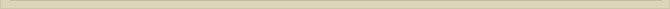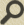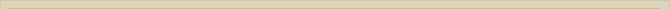## The Mechanical Free Rotator

When you are interested in physics you must read “Unbelievable“!

The atom is a so-called free rotator. The electrons rotating the nucleus infinitely at discrete energy levels without losing energy or collapsing. We consider first the mechanical free rotator.

Consider two masses Mp and Me circling around each other. Both masses are connected with a rigid mass less rod with length R=Rp+Re (figure 1).

When both masses Mp and Me are rotating and when there is no interaction with any other system the masses Mp and Me will rotate stable for infinite times.

Because both masses are connected with an imaginary rigid rod the dynamics can be described classical mechanics. The rotating point of the system (figure 1) is determined the relative masses, according to the following equations: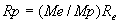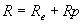Because both masses are rigidly connected to each other the following equations must be valid: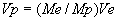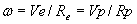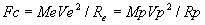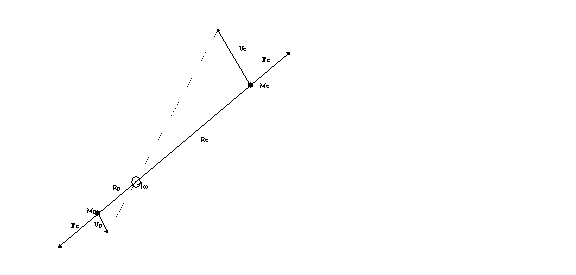Figure 1. The Mechanical Free Rotator

Next chapter: The EM-rotator